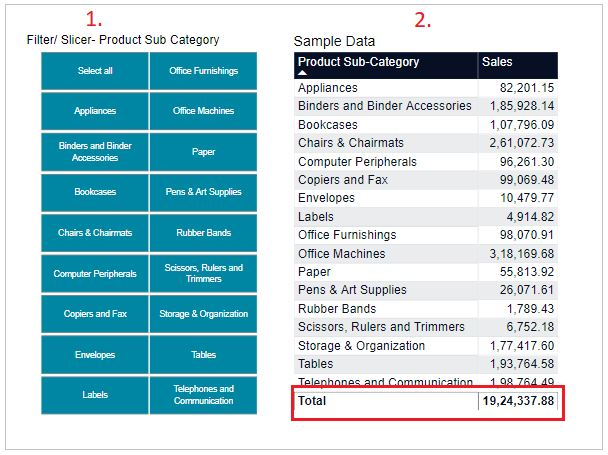Home » DAX » DAX – ALL function

# DAX – ALL functionAs it’s name suggests, Returns all the rows in a table, or all the values in a column. ALL function removes the applied filters from the filter context. Its comes under Filter function DAX category.

#### Syntax:

`ALL ({<table> or <column>, [<column>], [<column>] …})`

Refer similar DAX:-

#### Description:

 Sno. Parameter Description 1 table The table that you want to clear filters on. 2 column The column that you want to clear filters on.Power Bi DAX- ALL function Sample Data

So now, we will create to using DAX  ALL function and in that measure we will do sum of ‘Sales’ column.

```ALL_DAX =
CALCULATE(Sum(Orders[Sales]), ALL(Orders[Product Sub-Category]))```

We passed ‘Product sub category’ column under ALL function, so in that case whatever filters you apply, it will always returns sum of all ‘Product Sub Category‘ and that is 19,24,337.88 .Power Bi – ALL DAX function Example

As per above filters output screen, we did filter few Product sub categories but it ignores the filters and returned sum of total sales.

Refer similar DAX:-

Other DAX functions: DAX functions

## 7 thoughts on “DAX – ALL function”

1.Question… I want to find the MAX on a column and have the measure ignore any filters on the page. But writing this as “MAX(ALL(Table[Column]))” doesn’t see to work. Any ideas?

1.You can try this one , CALCULATE(MAX(Table[Column]),ALL(Table))

2.You can try this one CALCULATE(MAX(Table[Coloumn]), ALL(Table)

2.You can try this one CALCULATE(MAX(Table[Column]),ALL(Table))

3.But if you apply a region filter, the values change. So I understand that All function ignores only product subcategory filter, but it takes external filters. Am I right?

1.Yes, you are right, but if you want to apply filter on that table, then no need to give column name just give the table name, it will clear all the filters on that table.

2.Yes, you are right, but if you don’t want the values to be changed even after applying the external filters, then just remove the column name from dax and just give the table name, then it clears all the filters on that table.
eg: CALCULATE(SUM(Table[Column]),ALL(Table))